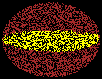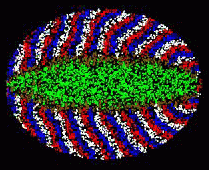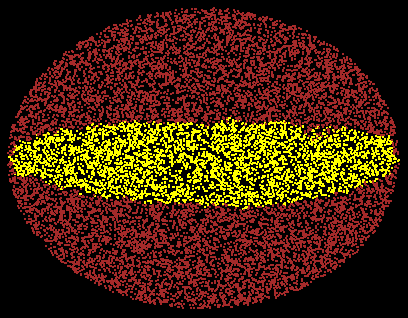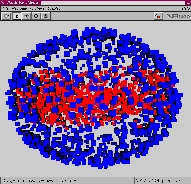A Day in the Life of a Tube Ron WeissThis page describes a simulation of the formation of a tube through the cooperation of a large number of independent elements with only local interactions. This is part of an attempt to understand how to program morphogenesis. Rather than precisely mimic the biological mechanisms, the focus here is to start with an approximation of the underlying assumptions about the operations of biological elements, and program them to produce similar results, i.e. form a complex biological entity using a programmatic interface. The elements are assumed to be distributed randomly and uniformly, and can only "communicate" with their neighbors.

Click below for an MPEG movie of the tube formation:

In this simulation, we model tube formation. The simulation environment consists of an embryo with 4 distinct element types: inner elements, embryo wall, a positively-charged end, and a negatively charged end. The goal here is to form a tube from the positive end to the negative end using only localized interactions between the elements. The following process achieves this goal:

 In the first phase, elements from the positive end initiate a signal that establishes the distance of all elements to the positive end. The signal propogates as a wave front. Initially, all elements assume they are infinitely far away from the positive end. When an element receives a distance measure from one of its neighbors, if the distance signifies that it is closer to the positive end than previously believed, it stores the new value, and locally broadcasts a message with a distance one greater than the value it received. After some time, all elements have a reasonable approximation of their distance from the positive end in terms of communication hops between elements.Phase 1

 In the second phase, the negative end initiates another wave to establish the distance of all elements from the negative end. The distance is computed in the same manner as above. In addition, the negative end also transmits its distance from the positive end.Phase 2

 While the distance wave propogates from the negative end, the tube begins to form. At this stage, elements know their distance from the positive end, their distance from the negative end, and the total distance between the two ends. Then, each element performs a simple computation that indicates whether it is approximately on the line between the two ends. If the distance from the positive end plus the distance from the negative end equals the total distance between the ends, then an element becomes part of the tube.Phase 3

 In the final stage, "peer pressure" is used to smooth out the tube to compensate for the imprecision in distance computations. All the inner elements broadcast whether they are part of the tube or not. If a tube element hears that a 3/4 majority of its neighbors are non-tube elements, then this element also becomes a non-tube element. Similarly, if a non-tube element hears that a 3/4 majority of its neighbors are tube elements, then it also becomse a tube element. The peer pressure process continues for several rounds.Final Tube

A 3D Simulation of Tube Formation

We also simulated tube formation in a three dimensional space. Below is a snapshot of the finished simulation. In blue are the wall elements, and in red are the tube elements.If you have a VRML enabled browser, click here to navigate through this vrml snapshot of the 3D simulation.